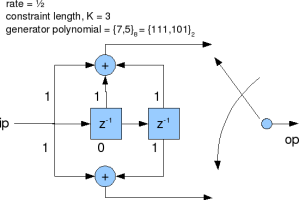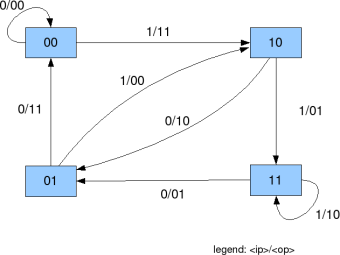- DSP log - http://www.dsplog.com -

Convolutional code

Posted By Krishna Sankar On January 4, 2009 @ 11:30 am In Coding | 40 Comments

Coding is a technique where redundancy is added to original bit sequence to increase the reliability of the communication. In this article, lets discuss a simple binary convolutional coding scheme at the transmitter and the associated Viterbi (maximum likelihood) decoding scheme at the receiver.

Update: For some reason, the blog is unable to display the article which discuss both Convolutional coding and Viterbi decoding. As a work around, the article was broken upto into two posts. This post descrbes a simple Binary Convolutional Coding scheme. For details on the Viterbi decoding algorithm, please refer to the post – Viterbi decoder. 

Chapter 8, Table 8.2-1 of Digital Communications by John Proakis  lists the various rate 1/2 convolutional coding schemes. The simplest among them has constraint length with generator polynomial . There are three parameters which define the convolotional code:

(a) Rate : Ratio of the number of input bits to the number of output bits. In this example, rate is 1/2 which means there are two output bits for each input bit.

(b) Constraint length : The number of delay elements in the convolutional coding. In this example, with there are two delay elements.

(c) Generator polynomial : Wiring of the input sequence with the delay elements to form the output. In this example, generator polynomial is . The output from the arm uses the XOR of the current input, previous input and the previous to previous input. The output from the uses the XOR of the current input and the previous to previous input.Figure 1: Convolutional code with Rate 1/2, K=3, Generator Polynomial [7,5] octal

From the Figure 1, it can be seen that the operation on each arm is like a FIR filtering (aka convolution) with modulo-2 sum at the end (instead of a normal sum). Hence the name Convolutional code.State transition

For understanding the Viterbi way of decoding the convolutional coded sequence, lets understand the relation between the input and output bits and the state transition.

 if ip = 0 if ip = 1 current state next state (op) next state (op) 00 00 (00) 10 (11) 01 00 (11) 10 (00) 10 01 (10) 11 (01) 11 01 (01) 11 (10)

Table 1: State transition table and the output valuesState transition for K=3, rate = 1/2 convolutional code

Figure 2: State transition for K=3, rate = 1/2 convolutional codeFor details on the Viterbi decoding algorithm, please refer to the post – Viterbi decoder. 

References

Tutorial on Convolutional Coding with Viterbi Decoding  – Mr. Chip Fleming

URL to article: http://www.dsplog.com/2009/01/04/convolutional-code/

URLs in this post:

 Viterbi decoder.: http://www.dsplog.com/2009/01/04/viterbi/

 Digital Communications by John Proakis: http://www.amazon.com/gp/redirect.html?ie=UTF8&location=http%3A%2F%2Fwww.amazon.com%2FDigital-Communications-John-Proakis%2Fdp%2F0072321113&tag=dl04-20&linkCode=ur2&camp=1789&creative=9325

 Tutorial on Convolutional Coding with Viterbi Decoding: http://home.netcom.com/~chip.f/Viterbi.html